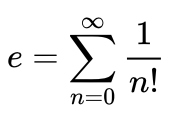# The value of e

## Problem

Create a function that computes an approximate value of the mathematical constant e using the following formula:This function should allow being called in one of the forms:

1. The argument defines the number of items in the formula: `e-approx(10)`.
2. If no argument is passed, the sum contains 100 elements: `e-approx()`.

Print the results for both cases.

## Example

``````\$ raku the-value-of-e.raku
2.7182818
2.718281828459045
``````